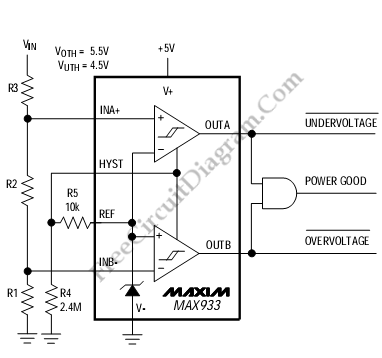# Window Detector for Under-/Over- Voltage DetectionThis is a Window Detector circuit. This circuit is used to detect over-voltage or under-voltage. This circuit uses the MAX933 and additional components like resistors. The over-voltage threshold of this circuit is 5.5V and the under-voltage threshold is 4.5V if we use the component values shown. We can choose different threshold by changing the values of R3, R2 and R1. Here is the circuit:This circuit has hysteresis which is added by R4 and R5 to prevent chatter at the output when the supply voltage is close to a threshold. OUTB and OUTA each provides an active-low over-voltage indication and an active-low under-voltage indication. An active high can be provided by ANDing the two outputs.

The design procedure is as follows :
1.Select the needed hysteresis and compute values for R5 and R4
2.Choose values of R1. The R1 values up to about 100Mohm can be used.
3.Compute R3 + R2
R3 + R2 = R1 (Voth/(Vref+Vh) – 1)
4.Compute R2
R2= (R1 + R2 + R3) x (Vref-Vh)/Vuth – R1
5.Compute R3
R3 = (R2 + R3) – R2
6.Verify the resistor values using following equations
Voth = (Vref + Vh) x (R1+R2+R3)/R1 = 5.474
Vuth = (Vref – Vh) x (R1+R2+R3)/(R1+R2) = 4.484
Vh = Vref x R5/R4

With Voth is overvoltage threshold, Vuth is undervoltage threshold,  and Vh is Hysteresis voltage. [Source: MAXIM Application Note]

The design procedure is as follows :

1. Select the needed hysteresis and compute values for R5 and R4

2. Choose values of R1. The R1 values up to about 100Mohm can be used.

3. Compute R3 + R2

R3 + R2 = R1 (Voth/(Vref+Vh) – 1)

4. Compute R2

R2= (R1 + R2 + R3) x (Vref-Vh)/Vuth – R1

5. Compute R3

R3 = (R2 + R3) – R2

6. Verify the resistor values using following equations

Voth = (Vref + Vh) x (R1+R2+R3)/R1 = 5.474

Vuth = (Vref – Vh) x (R1+R2+R3)/(R1+R2) = 4.484

Vh = Vref x R5/R4

With Voth is overvoltage threshold, Vuth is undervoltage threshold, and Vh is Hysteresis voltage.